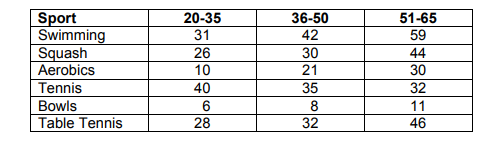# IMAT 2011 Q29 [Ages and Sports]

The table shows the number of people aged 20-35, 36-50 and 51-65 who participated in given sports at a leisure centre on a Sunday morning:In which of the other sports was the proportion of participants in the three age ranges closest to that for swimming?

A. Aerobics
B. Bowls
C. Squash
D. Table Tennis
E. Tennis

1 Like

Some Quick Questions to ask yourself when doing this type of problem solving:

• What is being asked? Underline the specifics in the question.
• Analyse the data, is there anything we can eliminate immediately?
• What information do I need to solve the question? Where can I find it?
• How can I draw or use the graph to help me?

This question provides us with some more difficult to manipulate numbers (not easy multiples of 5 to 10) which can seem discouraging. Always remember that even questions like this are meant to be solved quickly, so there are tricks and easy ways to do this. Start by using approximations and rounding the data to the nearest 5 or 10. It does not matter if your calculations are exact because you just have to approximate. Even some of the answers require a bit more conversions to solve, using approximations will eliminate the majority of answers.

Round the number and then simplify the ratio.

Swimming: 31:42:59 can be rounded to 30:40:60. We can then divide all of this by 10 to get 3:4:6. Now to compare with the rest.

A. Aerobics: 10:21:30 can be rounded to 10:20:30. We can then divide all of this by 10 to get 1:2:3.

B. Bowls: 6:8:11 can be rounded to 6:8:11. We can then divide all of this by 2 to get 3:4:5.5.

C. Squash: 26:30:44 can be rounded to 25:30:45. We can then divide all of this by 5 to get 5:6:9.

D. Table Tennis: 28:32:46 can be rounded to 30:30:45. We can then divide all of this by 15 to get 2:2:3.

E. Tennis: 40:35:32 can be rounded to 40:35:30. We can then divide all of this by 10 to get 4:3.5:3.

Notice how we got the approximate ratios without any crazy calculations. If you did this with a calculator, they would be roughly the same.

Comparing all the ratios, bowls is the closest to swimming, therefore B is the correct answer.